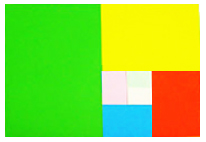# What's new## Why is A4 paper the size that it is?

, 23 October 2012Take a sheet of A4 paper and measure its sides. A4 is 210 millimetres wide and 297 millimetres long. It’s probably the most common size of paper and it’s used in most countries. However, A4 side lengths aren’t simple numbers like 200 or 300 millimetres. So why don’t we use something easier to measure?

If you take a sheet of paper and cut it halfway down the longer side, you end up with two new pieces of paper. These pieces of paper each have half the area of the original sheet, but they are the same proportions as the original sheet! There’s only one type of rectangle that has this ability. Because these half sheets have the same proportions as A4, they also have a name – A5.  If you cut an A5 sheet in half, you get two pieces of A6 paper, with the same proportions as A5 and A4. All these paper sizes are part of a set called the A series.

This pattern also works if you want to go bigger instead of smaller.  If you take two sheets of A4 paper and stick the long sides together, you’ll end up with a sheet of paper that has the same proportions as A4, but is twice as big. This size is called A3. You can use the same process to make A3 sheets into A2, and even A2 sheets into A1 paper.

So why is A4 paper called A4? A4 is half an A3, or one quarter of A2, but more importantly, it’s one sixteenth of A0. A0 has an area of one square metre (but it isn’t a square), and every other paper size in the A series is based on A0. We use A4 for writing on because it is a lot more convenient than trying to write on a square metre sheet of paper!

If you’re after more science news for kids, subscribe to Double Helix magazine!Categories:

## Similar posts

1.I love it, that is so interesting. I’m going to go put 16 A4 sheets together and measure the area!

2.Great piece of info?…keep it up

3.I did it, and it’s not 1 meter squared. Big disappointment when this site is intended for students.

1.Hi Winston,
A0 is not a square, but it is one square metre in size, or at least close to.
A0 is 841 mm wide and 1189 mm tall. That comes to 0.999949 square metres, which is as close as you can get without changing the shape of the paper or going to fractions of a millimetre. it is also almost exactly 16 times larger than an A4 sheet – four times wider and four times taller.

You can learn a bit more about the A series of paper sizes on Wikipedia:
https://en.wikipedia.org/wiki/ISO_216#A_series

Hope this helps!

4.You calculated incorrectly

1.Hi Luke,
I think my numbers are right? All the sizes are rounded to the nearest millimetre in the standard, which can make very small errors appear.

If there’s something clearly wrong, let me know exactly what the error is and I’ll address it.

5.Nah he said it was not one meter (sic) square but it is one square metre.
As David pointed out, the dims are 841 x 1189, same proportion as an A4 sheet, ie the long side is 1.4142135 times the length of the short side.
That number, 1.4142135 is the square root of 2, and that ratio is the magic reason why the A paper series “works” in maintaining the proportionality over the different sizes.

6.Cool!

7.Great article explaining why A4 paper is the size that it is! It’s fascinating to learn about the logic behind the A series paper sizes and how each size is a proportion of the A0 size. This system makes it incredibly versatile and adaptable for various uses. For those interested in more details about the A4 size and other paper sizes, you might find this resource helpful: https://papersdb.com/a/a4